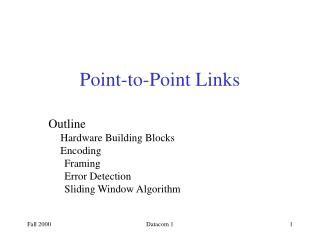Download PresentationOutline Hardware Building Blocks Encoding Framing Error Detection Sliding Window Algorithm

# Outline Hardware Building Blocks Encoding Framing Error Detection Sliding Window Algorithm - PowerPoint PPT PresentationDownload Presentation## Outline Hardware Building Blocks Encoding Framing Error Detection Sliding Window Algorithm

- - - - - - - - - - - - - - - - - - - - - - - - - - - E N D - - - - - - - - - - - - - - - - - - - - - - - - - - -
##### Presentation Transcript

1. Point-to-Point Links Outline Hardware Building Blocks Encoding Framing Error Detection Sliding Window Algorithm Datacom 1

2. Network Connections Memory latency is more important than CPU speed Datacom 1

3. Bits 0 0 1 0 1 1 1 1 0 1 0 0 0 0 1 0 NRZ Encoding Datacom 1

4. The Electromagnetic Spectrum Datacom 1

5. Cables and Fiber Link Bandwidths Datacom 1

6. Leased Line Service Bandwidth Datacom 1

7. Services at the home Datacom 1

8. Very high data rate DSL(symmetric) Datacom 1

10. Wireless LANs • HIPERLAN (High Performance European Radio LAN--5.2 GHz and 17 GHz • IEEE 802.11---2.4 GHz • PICONET • Bluetooth (Ericsson, Nokia, IBM, Toshiba, Intel) • 2.45 GHz, 1 Mbps, 10 M Datacom 1

11. Problem: Consecutive 1s or 0s • Low signal (0) may be interpreted as no signal • High signal (1) leads to baseline wander • Unable to recover clock Datacom 1

12. Alternative Encodings • Non-return to Zero Inverted (NRZI) • make a transition from current signal to encode a one; stay at current signal to encode a zero • solves the problem of consecutive ones • Manchester • transmit XOR of the NRZ encoded data and the clock • only 50% efficient. Datacom 1

13. Coding Examples Datacom 1

14. Encodings (cont) • 4B/5B • every 4 bits of data encoded in a 5-bit code • 5-bit codes selected to have no more than one leading 0 and no more than two trailing 0s • thus, never get more than three consecutive 0s • resulting 5-bit codes are transmitted using NRZI • achieves 80% efficiency Datacom 1

15. Bits Node A Adaptor Adaptor Node B Frames Framing • Break sequence of bits into a frame • Typically implemented by network adaptor Datacom 1

16. 8 16 16 8 Beginning Ending Header Body CRC sequence sequence Approaches • Bit-Oriented Approach-High level Data Link Control (HDLC) • delineate frame with special pattern: 01111110 • problem: special pattern appears in the payload • solution: bit stuffing • sender: insert 0 after five consecutive 1s • receiver: delete 0 that follows five consecutive 1s Datacom 1

17. Bit stuffing example Datacom 1

18. Approaches (cont) • Byte oriented Protocols • Byte Counting- based • include payload length in header • e.g., Digital Data Communication Message Protocol (DDCMP) • problem: count field corrupted • solution: catch when CRC fails Datacom 1

19. Byte stuffing example Datacom 1

20. Approaches (cont) • Clock-based • each frame is 125us long • e.g., SONET: Synchronous Optical Network • STS-n (STS-1 = 51.84 Mbps) Datacom 1

21. Error Detection and Correction • Two dimensional Parity • Internet checksum • Cyclic Redundency Check Datacom 1

22. Two Dimensional Parity Datacom 1

23. Cyclic Redundancy Check • Add k bits of redundant data to an n-bit message • want k << n • e.g., k = 32 and n = 12,000 (1500 bytes) • Represent n-bit message as n-1 degree polynomial • e.g., MSG=10011010 as M(x) = x7 + x4 + x3 + x1 • Let k be the degree of some divisor polynomial • e.g., C(x) = x3 + x2 + 1 Datacom 1

24. CRC (cont) • Transmit polynomial P(x) that is evenly divisible by C(x) • shift left k bits, i.e., M(x)xk • subtract remainder of M(x)xk / C(x) from M(x)xk • Receiver polynomial P(x) + E(x) • E(x) = 0 implies no errors • Divide (P(x) + E(x)) by C(x); remainder zero if: • E(x) was zero (no error), or • E(x) is exactly divisible by C(x) Datacom 1

25. Selecting C(x) • All single-bit errors, as long as the xk and x0 terms have non-zero coefficients. • All double-bit errors, as long as C(x) contains a factor with at least three terms • Any odd number of errors, as long as C(x) contains the factor (x + 1) • Any ‘burst’ error (i.e., sequence of consecutive error bits) for which the length of the burst is less than k bits. • Most burst errors of larger than k bits can also be detected • See Table 2.6 on page 102 for common C(x) Datacom 1

26. CRC Example Datacom 1

27. CRC Example Datacom 1

28. CRC Example Datacom 1

29. CRC Example Datacom 1

30. Internet Checksum Algorithm • View message as a sequence of 16-bit integers; sum using 16-bit ones-complement arithmetic; take ones-complement of the result. u_short cksum(u_short *buf, int count) { register u_long sum = 0; while (count--) { sum += *buf++; if (sum & 0xFFFF0000) { /* carry occurred, so wrap around */ sum &= 0xFFFF; sum++; } } return ~(sum & 0xFFFF); } Datacom 1